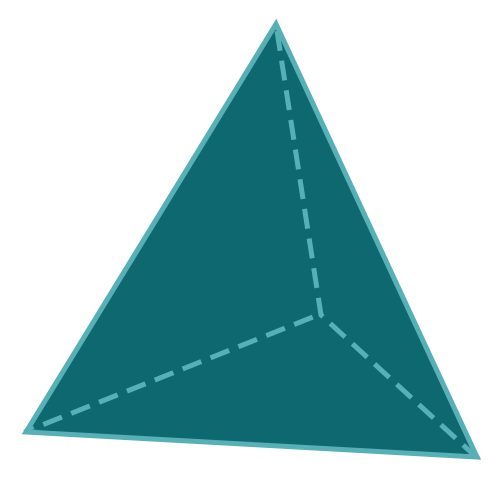Report a Problem
Suggestions

#Calculate The Volume of A Tetrahedron Pyramid

Last updated: Saturday, June 24, 2023
Select a type of pyramid below
Rectangular Base
Square Base
Equilateral Triangular Base
Tetrahedron
Pentagonal Base
Hexagonal Base

A tetrahedron pyramid is a polyhedron composed of four triangular faces, four vertices, and six edges. It is the simplest of all pyramids and is also known as a triangular pyramid. Each face is an equilateral triangle, and its symmetrical shape gives it unique properties in mathematics and geometry.

The tetrahedron pyramid appears in various real-life scenarios, such as the construction of bridges and towers that utilize its inherent stability and strength. In the field of chemistry, the shape represents the molecular geometry of certain compounds, like methane, due to its optimal spatial arrangement.

Easily calculate the volume of a tetrahedron pyramid with step-by-step guidance using our free calculator below.

The formula for determining the volume of a tetrahedron pyramid is defined as:
$$V$$ $$=$$ $$\dfrac{a^3}{6 \cdot \sqrt{2}}$$
$$V$$: the volume of the pyramid
$$a$$: the length of any side
The SI unit of volume is: $$cubic \text{ } meter\text{ }(m^3)$$

## Find $$V$$

Use this calculator to determine the volume of a tetrahedron when the length of any side is given.
Hold & Drag
CLOSE
the length of any side
$$a$$
$$meter$$
Bookmark this page or risk going on a digital treasure hunt again